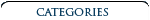3-View Scale Airplane Drawing Books & CDs Aero Albums Books Air Force Legends Air Racing Books Airliner Books Aviation CDs Aviation Century Series Biographicals British Aviation Books Children's Aviation Books Civil Aviation Books A-G Civil Aviation Books H-N Civil Aviation Books O-S Civil Aviation Books T-Z DVDs & Videos Early Aviation Books Golden Age Era Golden Age Of Aviation Series Military Aviation Modeling & R/C Airplane Books Naval Fighters Navy Books Nose Art Books Paul Matt Individual 3-View Drawings Personal Adventures Post World War II Books Soaring, Ultralights & Ballooning Time-Life's Epic Of Flight Series Type Airplane Books A-G Type Airplane Books H-P Type Airplane Books R-Z World War I Aviation Books World War II Aviation Books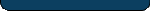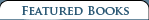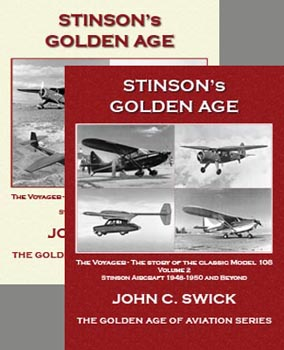Stinson's Golden Age - Vol 1 & Vol 2 \$ 50.00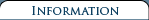Shipping and Returns   Terms and Conditions  Privacy Policy  Contact Us### Bonanza Around the World

 Retail Price: \$14.50 By: Dennis Stewart In 1994 the Author embarked on an around-the-world air race with several other private aircraft from a variety of countries in a race sponsored by Arc en Ciel. He flew as navigator and copilot with owner Bob Reiss in an A36 Beechcraft Bonaza prop-jet. Some crews were serious racers, others participated for the sheer adventure of the journey вЂ” long over-water crossings, personal risks in hostile political situations and cultural differences.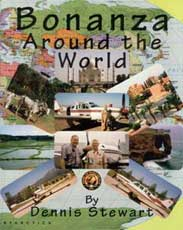No one anticipated seeing the stark human suffering in India nor the racism in Vietnam. The book is clearly written and Mr. Stewart pulls no punches in describing what they saw and experiences they had.

This was an extraordinary journey where strange alliances developed and political experiences were contradicted: professional and courteous Iranian controllers, contrasted with being denied access to Saudi Arabian airspace.

This is also the story of a remarkable and reliable aircraft, superbly engineered. The Author describes in detail the preparations for such a flight, and the many in-flight challenges they faced.

Bonanza Around the World
Book Contents

ChapterВ В В В В В В В В В В В В В В В В В В В В В В В В В В В В В В В В В В В В В В В В В В В В В В В В В В В В В В В В В В В В В В В В В В В В В В В  Page

1В В В В В В В В В  PreparationsВ В В В В В В В В В В В В В В В В В В В В В В В В В В В В В В В В В В В В В В В В В В В В В В В В В В В В В В В В В В В В В В В В В В В В В В В В В В В  1
2В В В В В В В В В  The Journey BeginsВ В В В В В В В В В В В В В В В В В В В В В В В В В В В В В В В В В В В В В В В В В В В В В В В В В В В В В В В В В В В В В В В В В  13
3В В В В В В В В В  Montreal to St. JohnвЂ™sВ В В В В В В В В В В В В В В В В В В В В В В В В В В В В В В В В В В В В В В В В В В В В В В В В В В В В В В В В В В В В  25
4В В В В В В В В В  St. JohnвЂ™s to MarrakechВ В В В В В В В В В В В В В В В В В В В В В В В В В В В В В В В В В В В В В В В В В В В В В В В В В В В В В В В В В  31
5В В В В В В В В В  Marrakech to IstanbulВ В В В В В В В В В В В В В В В В В В В В В В В В В В В В В В В В В В В В В В В В В В В В В В В В В В В В В В В В В В В В В  41
6В В В В В В В В В  Istanbul to DubaiВ В В В В В В В В В В В В В В В В В В В В В В В В В В В В В В В В В В В В В В В В В В В В В В В В В В В В В В В В В В В В В В В В В В В В  49
7В В В В В В В В В  Dubai to AgraВ В В В В В В В В В В В В В В В В В В В В В В В В В В В В В В В В В В В В В В В В В В В В В В В В В В В В В В В В В В В В В В В В В В В В В В В В В  57
8В В В В В В В В В  Agra to Ho Chi Minh City (Saigon)В В В В В В В В В В В В В В В В В В В В В В В В В В В В В В В В В В В В В В В В В В  67
9В В В В В В В В В  Saigon to NahaВ В В В В В В В В В В В В В В В В В В В В В В В В В В В В В В В В В В В В В В В В В В В В В В В В В В В В В В В В В В В  77
10В В В В В В В  Naha to SendaiВ В В В В В В В В В В В В В В В В В В В В В В В В В В В В В В В В В В В В В В В В В В В В В В В В В В В В В В В В В В В  83
11В В В В В В В  Sendai to PetropavlovskВ В В В В В В В В В В В В В В В В В В В В В В В В В В В В В В В В В В В В В В В В В В В В В В В В В В В В В В В В В  91
12В В В В В В В  Petropavlovsk to AnchorageВ В В В В В В В В В В В В В В В В В В В В В В В В В В В В В В В В В В В В В В В В В В В В В В В В В В В  101
13В В В В В В В  Anchorage to SitkaВ В В В В В В В В В В В В В В В В В В В В В В В В В В В В В В В В В В В В В В В В В В В В В В В В В В В В В В В В В В В В В В В В В  107
14В В В В В В В  Sitka to EugeneВ В В В В В В В В В В В В В В В В В В В В В В В В В В В В В В В В В В В В В В В В В В В В В В В В В В В В В В В В В В В В В В В В В В В В В В  113
15В В В В В В В  Eugene to San DiegoВ В В В В В В В В В В В В В В В В В В В В В В В В В В В В В В В В В В В В В В В В В В В В В В В В В В В В В В В В В В В В В В  115
EpilogueВ В В В В В В В В В В В В В В В В В В В В В В В В В В В В В В В В В В В В В В В В В В В В В В В В В В В В В В В В В В В В В В В В В В В В В В В В В В В В В В В В В В В В В В В В В В В В В  117

121 pages, SB, 8ВЅ x 11, 110 photos & illustrations.

### Bonanza Around the World

#### Item Number: 96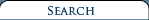Type in the title of book or similar words to help find your item(s).